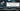# Longest Palindrome Substring - Leet Code Solution

August 27, 2019## Problem Statement

Given a string s, find the longest palindromic substring in s. You may assume that the maximum length of s is 1000.

``````Example 1:

Output: "bab"
Note: "aba" is also a valid answer.

Example 2:

Input: "cbbd"
Output: "bb"``````

## Brute Force Solution

• Find all substrings of a string
• Check if that substring is a palindrome string
• Keep a track of longest palindrome string found so far

Simple!

### Code

``````public class Q5_LongestPalindromeSubstring_Simple {
private String str;

public Q5_LongestPalindromeSubstring_Simple(String str) {
this.str = str;
}

private boolean isPalindrome(String s) {
int lastIndex = s.length()-1;
int startIndex = 0;
while (startIndex < s.length() && lastIndex >= 0) {
if (s.charAt(startIndex) != s.charAt(lastIndex)) {
return false;
}
startIndex ++;
lastIndex --;
}
return true;
}

public String longestPalindrome() {
String max = "";

//generate all substrings
for (int i=0; i<this.str.length(); i++) {
for (int j=i+1; j<this.str.length(); j++) {
String substring = this.str.substring(i, j);
if (this.isPalindrome(substring)) {
if (max.length() < substring.length()) {
max = substring;
}
}
}
}

return max;
}
}``````

### Runtime Complexity

It is O(n^3)

• O(n^2) in find all substrings
• For every substring, find out if it is a palindrome - O(n)

## Optimized Solution

For optimized solutions, we should go deeper into the requirements. What is palindrome. It can be found in two ways:

• Centered around a single character. Length will be odd. Example: abc
• Centered around two characters. Length will be even. Example: abba

The solution is around starting from the one element, and try to expand it from both sides. Expand it till you find same character.

### Algorithm

• Iterate over string
• Try to expand it in two ways. Around single character and around two characters
• Keep track of max found palindrome substring

### Code

``````public class Q5_LongestPalindromeSubstring_Optimized {
private String str;

public Q5_LongestPalindromeSubstring_Optimized(String str) {
this.str = str;
}

//expanding around elements passed
private String findPalindrome(int startIndex, int endIndex) {
String palindrome = "";
while (startIndex >= 0 && endIndex < this.str.length() && this.str.charAt(startIndex) == this.str.charAt(endIndex)) {
palindrome = this.str.substring(startIndex, endIndex+1);
startIndex --;
endIndex ++;
}

return palindrome;
}

public String longestPalindrome() {
String max = "";

for (int i=0; i<this.str.length()-1; i++) {
String s1 = this.findPalindrome(i, i);
String s2 = this.findPalindrome(i,  i+1);

String maxOfTwo = s1.length() > s2.length() ? s1 : s2;
if (max.length() < maxOfTwo.length()) {
max = maxOfTwo;
}
}

return max;
}
}
``````